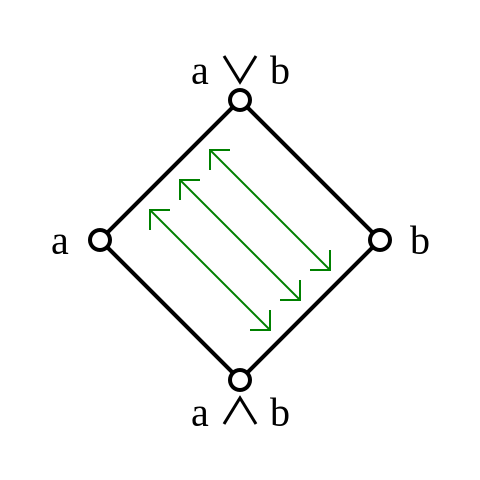## September 30, 2015

### An Exact Square from a Reedy Category

#### Posted by Emily RiehlI first learned about exact squares from a blog post written by Mike Shulman on the $n$-Category Café.

Today I want to describe a family of exact squares, which are also homotopy exact, that I had not encountered previously. These make a brief appearance in a new preprint, A necessary and sufficient condition for induced model structures, by Kathryn Hess, Magdalena Kedziorek, Brooke Shipley, and myself.

Proposition. If $R$ is any (generalized) Reedy category, with $R^+ \subset R$ the direct subcategory of degree-increasing morphisms and $R^- \subset R$ the inverse subcategory of degree-decreasing morphisms, then the pullback square: $\array{ iso(R) & \to & R^- \\ \downarrow & \swArrow id & \downarrow \\ R^+ & \to & R}$ is (homotopy) exact.

In summary, a Reedy category $(R,R^+,R^-)$ gives rise to a canonical exact square, which I’ll call the Reedy exact square.

Posted at 8:50 PM UTC | Permalink | Followups (9)

## September 19, 2015

### The Free Modular Lattice on 3 Generators

#### Posted by John BaezThe set of subspaces of a vector space, or submodules of some module of a ring, is a lattice. It’s typically not a distributive lattice. But it’s always modular, meaning that the distributive law

$a \vee (b \wedge c) = (a \vee b) \wedge (a \vee c)$

holds when $a \le b$ or $a \le c$. Another way to say it is that a lattice is modular iff whenever you’ve got $a \le a'$, then the existence of an element $x$ with

$a \wedge x = a' \wedge x \; \mathrm{and} \; a \vee x = a' \vee x$

is enough to imply $a = a'$. Yet another way to say it is that there’s an order-preserving map from the interval $[a \wedge b,b]$ to the interval $[a,a \vee b]$ that sends any element $x$ to $x \vee a$, with an order-preserving inverse that sends $y$ to $y \wedge b$:Dedekind studied modular lattices near the end of the nineteenth century, and in 1900 he published a paper showing that the free modular lattice on 3 generators has 28 elements.

One reason this is interesting is that the free modular lattice on 4 or more generators is infinite. But the other interesting thing is that the free modular lattice on 3 generators has intimate relations with 8-dimensional space. I have some questions about this stuff.

Posted at 9:57 PM UTC | Permalink | Followups (78)

## September 14, 2015

### Where Does The Spectrum Come From?

#### Posted by Tom LeinsterPerhaps you, like me, are going to spend some of this semester teaching students about eigenvalues. At some point in our lives, we absorbed the lesson that eigenvalues are important, and we came to appreciate that the invariant par excellence of a linear operator on a finite-dimensional vector space is its spectrum: the set-with-multiplicities of eigenvalues. We duly transmit this to our students.

There are lots of good ways to motivate the concept of eigenvalue, from lots of points of view (geometric, algebraic, etc). But one might also seek a categorical explanation. In this post, I’ll address the following two related questions:

1. If you’d never heard of eigenvalues and knew no linear algebra, and someone handed you the category $\mathbf{FDVect}$ of finite-dimensional vector spaces, what would lead you to identify the spectrum as an interesting invariant of endomorphisms in $\mathbf{FDVect}$?

2. What is the analogue of the spectrum in other categories?

I’ll give a fairly complete answer to question 1, and, with the help of that answer, speculate on question 2.

(New, simplified version posted at 22:55 UTC, 2015-09-14.)

Posted at 1:06 AM UTC | Permalink | Followups (37)

## September 3, 2015

### Rainer Vogt

#### Posted by Tom LeinsterI was sad to learn that Rainer Vogt died last month. He is probably best-known to Café readers for his work on homotopy-algebraic structures, especially his seminal 1973 book with Michael Boardman, Homotopy Invariant Algebraic Structures on Topological Spaces.

It was Boardman and Vogt who first studied simplicial sets satisfying what they called the “restricted Kan condition”, later renamed quasi-categories by André Joyal, and today (thanks especially to Jacob Lurie) the most deeply-explored incarnation of the notion of $(\infty, 1)$-category. Their 1973 book also asked and answered fundamental questions like this:

Given a topological group $X$ and a homotopy equivalence between $X$ and another space $Y$, what structure does $Y$ acquire?

Clearly $Y$ is some kind of “group up to homotopy” — but the details take some working out, and Boardman and Vogt did just that.

Martin Markl wrote a nice tribute to Vogt, which I reproduce with permission here:

Posted at 11:54 PM UTC | Permalink | Followups (5)

## September 2, 2015

### How Do You Handle Your Email?

#### Posted by Tom LeinsterThe full-frontal assault of semester begins in Edinburgh in twelve days’ time, and I have been thinking hard about coping strategies. Perhaps the most incessantly attention-grabbing part of that assault is email.

Reader: how do you manage your email? You log on and you have, say, forty new mails. If your mail is like my mail, then that forty is an unholy mixture of teaching-related and admin-related mails, with a relatively small amount of spam and perhaps one or two interesting mails on actual research (at grave peril of being pushed aside by the rest).

So, you’re sitting there with your inbox in front of you. What, precisely, do you do next?

Posted at 12:47 PM UTC | Permalink | Followups (24)